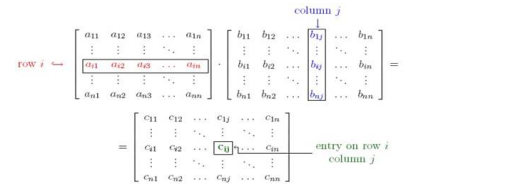# Solve this following`
Question:

Compute $\mathrm{AB}$ and BA, which ever exists when

$A=\left[\begin{array}{cc}2 & -1 \\ 3 & 0 \\ -1 & 4\end{array}\right]$ and $B=\left[\begin{array}{cc}-2 & 3 \\ 0 & 4\end{array}\right]$

Solution:

Given : $A=\left[\begin{array}{cc}2 & -1 \\ 3 & 0 \\ -1 & 4\end{array}\right]$ and $B=\left[\begin{array}{cc}-2 & 3 \\ 0 & 4\end{array}\right]$

Matrix A is of order $3 \times 2$, and Matrix $B$ is of order $2 \times 2$

To find: matrix $\mathrm{AB}$ and $\mathrm{BA}$

Formula used:Where $c_{i j}=a_{i 1} b_{1 j}+a_{i 2} b_{2 j}+a_{i 3} b_{3 j}+\ldots \ldots \ldots \ldots \ldots .+a_{i n} b_{n j}$

If $A$ is a matrix of order $a \times b$ and $B$ is a matrix of order $c \times d$, then matrix $A B$ exists and is of order $a \times d$, if and only if $b=$ $C$

If $A$ is a matrix of order $a \times b$ and $B$ is a matrix of order $c \times d$, then matrix $B A$ exists and is of order $c \times b$, if and only if $d=$ a

For matrix $\mathrm{AB}, \mathrm{a}=3, \mathrm{~b}=\mathrm{c}=2, \mathrm{~d}=2$, thus matrix $\mathrm{AB}$ is of order $3 \times 2$

Matrix $A B=\left[\begin{array}{cc}2 & -1 \\ 3 & 0 \\ -1 & 4\end{array}\right] \times\left[\begin{array}{cc}-2 & 3 \\ 0 & 4\end{array}\right]=\left[\begin{array}{cc}2(-2)+(-1)(0) & 2(3)+(-1)(4) \\ 3(-2)+0(0) & 3(3)+0(4) \\ -1(-2)+4(0) & -1(3)+4(4)\end{array}\right]$

Matrix $A B=\left[\begin{array}{cc}-4+0 & 6-4 \\ -6+0 & 9+0 \\ 2+0 & -3+16\end{array}\right]=\left[\begin{array}{cc}-4 & 2 \\ -6 & 9 \\ 2 & 13\end{array}\right]$

Matrix $A B=\left[\begin{array}{cc}-4 & 2 \\ -6 & 9 \\ 2 & 13\end{array}\right]$

Matrix $A B=\left[\begin{array}{cc}-4 & 2 \\ -6 & 9 \\ 2 & 13\end{array}\right]$

For matrix BA, a = 3,b = c = 2,d = 2 ,thus matrix BA exists, if and only if d=a

But $3 \neq 2$

Thus matrix BA does not exist